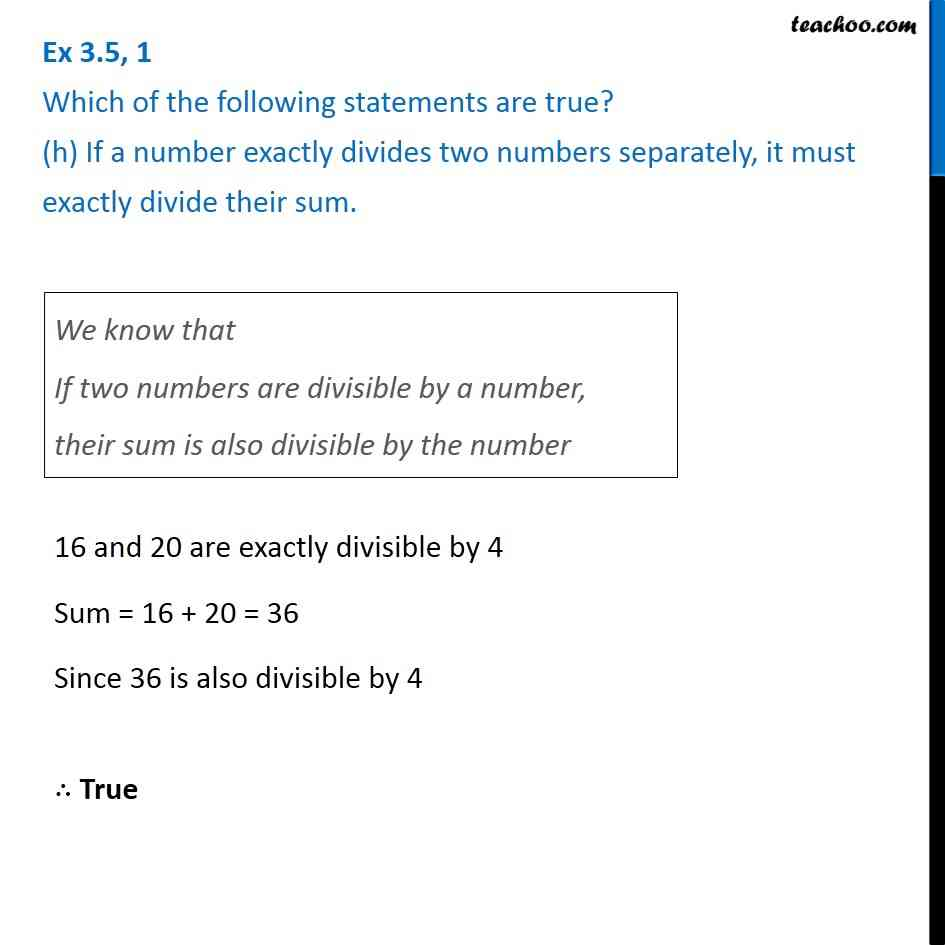Ex 3.5

Chapter 3 Class 6 Playing with Numbers
Serial order wiseLearn in your speed, with individual attention - Teachoo Maths 1-on-1 Class

### Transcript

Ex 3.5, 1 Which of the following statements are true? (h) If a number exactly divides two numbers separately, it must exactly divide their sum.We know that If two numbers are divisible by a number, their sum is also divisible by the number 16 and 20 are exactly divisible by 4 Sum = 16 + 20 = 36 Since 36 is also divisible by 4 ∴ True